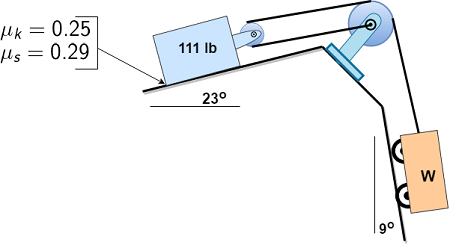# Determine the range of weights W for which the 111-lb block is in equilibrium. All wheels and...

## Question:

Determine the range of weights W for which the 111-lb block is in equilibrium. All wheels and pulleys have negligible friction.## Coefficient of Friction:

When a body is in state of rest position, then the coefficient of static friction will act on the body. When the body is in the state of movement there is also force of friction on the body, in this situation the coefficient of kinetic friction will act on the body.

Become a Study.com member to unlock this answer! Create your account

Given data:

• The weight of block is: {eq}{W_b} = 111\;{\rm{lb}} {/eq}
• The coefficient of kinetic friction between the surface and block is: {eq}{\mu...Friction: Definition and Types

from

Chapter 7 / Lesson 5
39K

You experience friction all the time, and you should be glad you do! Friction helps keep stationary objects in place as well as slow moving objects down as they slide across a surface. This lesson identifies what friction is and explains the two ways we find this force on earth.